# The diagonals 2

The diagonals AC and BD of a rectangle ABCD intersect each other at a point O. If OA=4cm, find AC and BD.

AC =  8 cm
BD =  8 cm

### Step-by-step explanation: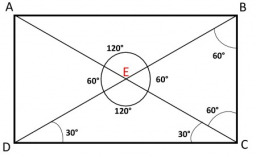Did you find an error or inaccuracy? Feel free to write us. Thank you!Dr Math
Hint: The diagonals bisect each other. The diagonals of the rectangle are equal.Dr Math
hanka
HANNKA
ZARKA
ZARA
DASATips to related online calculators
Do you want to convert length units?

#### You need to know the following knowledge to solve this word math problem:

We encourage you to watch this tutorial video on this math problem:

## Related math problems and questions:

• Rhombus constructionConstruct ABCD rhombus if its diagonal AC=9 cm and side AB = 6 cm. Inscribe a circle in it touching all sides...
• Parallerogram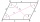In parallelogram ABCD is a = 2.5 cm and height to side a is 7.2 cm and height to side b is 4 cm. Find the side length b.
• Rhombus ABCDRhombus ABCD, |AC| = 90 cm, |BD| = 49 cm. Calculate the perimeter of the rhombus ABCD.
• The corridor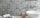The corridor is 12 m long and 3.6 m wide. It must be paved with rectangular tiles measuring 15 cm and 30 cm. Are 1000 pieces of tiles enough to pave the corridor?
• Ratio of perimeters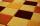Rectangle ABCD has dimensions 3 cm and 4 cm, KLMN rectangle has dimensions 4 cm and 12 cm. Calculate the ratio of the perimeter ABCD and perimeter KLMN.
• Coordinates of the intersection of the diagonals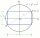In the rectangular coordinate system, a rectangle ABCD is drawn. The vertices of the rectangle are determined by these coordinates A = (2.2) B = (8.2) C = (8.6) D = (2.6) Find the coordinates of the intersection of the diagonals of the ABCD rectangle
• Colored paperCan a 4 cm, 3 cm and 2 cm block be glued with a colored quarter of paper measuring 7 cm and 8 cm?
• Diagonal intersectisosceles trapezoid ABCD with length bases | AB | = 6 cm, CD | = 4 cm is divided into 4 triangles by the diagonals intersecting at point S. How much of the area of the trapezoid are ABS and CDS triangles?
• Area and perimeter 2Find the area and the perimeter of a rectangle of length 45 1/2 cm and breadth 16 2/3 cm.
• Trapezium diagonalsIt is given trapezium ABCD with bases | AB | = 12 cm, |CD| = 8 cm. Point S is the intersection of the diagonals for which |AS| is 6 cm long. Calculate the length of the full diagonal AC.
• Trapezoid ABCDABDC is a trapezoid in which AB and CD are parallel sides measuring 6 and 9, respectively. Angles ABC and BCD are both right angles. Find the length of segment BD.
• Diagonals in diamons/rhombusRhombus ABCD has side length AB = 4 cm and a length of one diagonal of 6.4 cm. Calculate the length of the other diagonal.
• The bookThe book has 280 pages. Each side is a rectangle with sides 15 cm and 22 cm. a) how many leaves has the book? b) at least how many square meters of paper should be used to production this book?
• Rectangle 45The perimeter of a rectangle is 60cm. If the length of the rectangle is 20cm. a)find the width b)find the area.
• Picture 2Picture free is of length 14.2 cm and breadth 10.4 cm. The frame has border of uniforms with 2.6 cm. Find the area of photograph.
• Second sideCalculate the length of the other side of the rectangle if its circumference is 60 cm and one side is 10 cm long.
• Prism 4 sidesFind the surface area and volume four-sided prism high 10cm if its base is a rectangle measuring 8 cm and 1.2dm